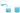# Density Calculator

## Density

Mass

Kg

Volume

m3

Density

kg/m3### Density

Density is a measurement that relates the quantity of matter an object has to its volume.

### Density Formula

Density is resolved by dividing the mass of an object by its volume.
density = mass (of the object) / volume
Calculate Density by entering two inputs to find the third.